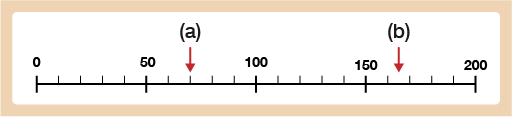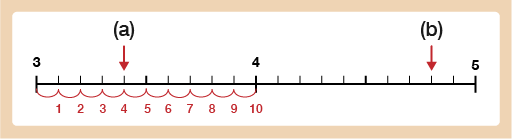Everyday maths 2 (Wales)

Start this free course now. Just create an account and sign in. Enrol and complete the course for a free statement of participation or digital badge if available.

# 5.1 Scale examples

Take a look at the following examples.Figure 23 Scale with numbered intervals of 50

You can see that this scale is marked up in numbered intervals of 50. However, what does each line in between each numbered interval represent? You can use your judgement to help you to figure out what each small step represents.

Watch this video (https://corbettmaths.com/ 2013/ 04/ 27/ reading-scales/ [Tip: hold Ctrl and click a link to open it in a new tab. (Hide tip)] ) for further information about how to do this.

Alternatively, you can work this out using division. If you count on from 0 to 50 on this scale, there are 5 steps: 50 ÷ 5 = 10, so each step is 10.

• a.The arrow is pointing to the 2nd mark after 50. As the steps are going up in 10s, the arrow is pointing to 70.
• b.The arrow is halfway between the 1st and 2nd step after 150. The first step is 160 and the second is 170 so the arrow is pointing to 165.

Sometimes you will need to read scales where the reading will be a decimal number.Figure 24 Scale with single numbered intervals going from 3–5

If you look at this scale, it goes up in numbered intervals of 1. From one whole number to the next whole number there are 10 small steps. 1 ÷ 10 = 0.1, so each step is 0.1.

Hint: Have a look at the image to show how to count the number of steps between the numbered markers.

• a.The arrow is pointing to the fourth step after 3 so the arrow is pointing to 3.4.
• b.The arrow is pointing to the eighth step after 4, so the arrow is pointing to 4.8.#### Find out more

*Eligibility rules apply for financial support.